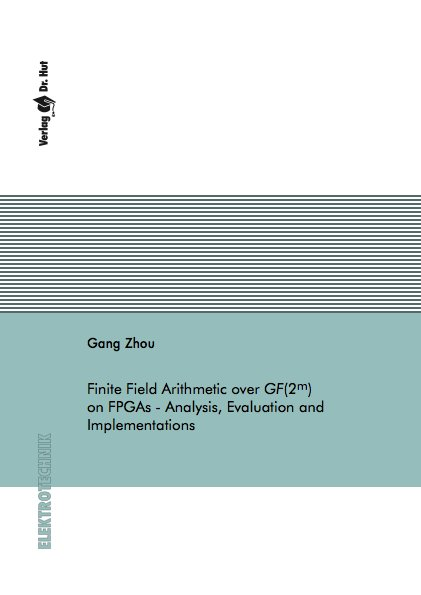Datenbestand vom 20. August 2019aktualisiert am 20. August 2019

# ISBN 9783843921503Euro 84,00 inkl. 7% MwSt

Gang Zhou
Finite Field Arithmetic over GF(2 to m) on FPGAs - Analysis, Evaluation and Implementations

179 Seiten, Dissertation Technische Universität Braunschweig (2014), Softcover, A5

## Zusammenfassung / Abstract

Finite field arithmetic is a central aspect of modern cryptographic systems. Complexity, architectures and implementations for finite field arithmetic over GF(2 to m) are extensively studied in recent years. Most previous work is designed for ASIC implementations, where AND/XOR/NAND gates are used as primitives. For various reasons (e.g. fast erasability), the use of FPGAs for cryptographic applications becomes more and more attractive. However most existed FPGA implementations are still gate-oriented. The gate-oriented designs are synthesized and mapped into technology dependent designs with FPGA primitives, e.g., Look-Up-Tables (LUTs), by synthesis tools.

Within this thesis the complexity analysis of finite field arithmetic is directly based on LUTs, which are the most important primitives in FPGAs. The analysis provides early resource and performance estimation on FPGAs in the design process. The LUT-based analysis also delivers efficient architectures, which are different to the gate-oriented architectures. The influences of synthesis tools are excluded as well.

The main part of this thesis focuses on the complexity analysis of finite field multipliers. The complexity analysis for both quadratic and sub-quadratic finite field multipliers is discussed in detail. Explicit formulae for the number of gates and the number of LUTs are presented. The analysis is validated by abundant experimental results. For quadratic multipliers, bit serial, bit parallel and digit serial multipliers are studied. Optimum digit sizes are revealed. For sub-quadratic Karatsuba-Ofman multipliers (KOM), this thesis introduces new complexity formulae for odd-term polynomials. Combined with analysis for even-term polynomials, the complexity of arbitrary bit depth (often prime number) is derived. It is shown that optimum constructions of KOM differ for different technologies, i.e., in ASICs, on 4-input-LUT FPGAs and 6-input-LUT FPGAs. By revealing the common expression in the degree alignment stage of KOM, a new lower gate upper-bound is reached. With the help of LUT-based analysis, efficient implementations of finite field multipliers on FPGAs are presented. The extension of KOM to unbalanced operand sizes is presented as well.

The LUT-based complexity analysis is extended for finite field division and AES implementations as well. Efficient and high throughput AES-GCM implementations on Xilinx Virtex-4 and Virtex-5 FPGAs are presented.